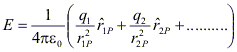# Electric field

## 6. Electric Field

• Electrical interaction between charged particles can be reformulated using the concept of electric field.

• To understand the concept consider the mutual repulsion of two positive charged bodies as shown in fig (a)• Now if remove the body B and label its position as point P as shown in fig (b), the charged body A is said to produce an electric field at that point (and at all other points in its vicinity)

• When a body B is placed at point P and experiences force F, we explain it by a point of view that force is exerted on B by the field not by body A itself.

• The body A sets up an electric field and the force on body B is exerted by the field due to A.

• An electric field is said to exists at a point if a force of electric origin is exerted on a stationary charged (test charge) placed at that point.

• If F is the force acting on test charge q placed at a point in an electric field then electric field at that point is
E = F/q
or F = qE

• Electric field is a vector quantity and since F = qE the direction of E is the direction of F.

• Unit of electric field is (N.C-1)

Q. Find the dimensions of electric field
Ans. [MLT-3A-1]

## 7. Calculation of Electric Field

• In previous section we studied a method of measuring electric field in which we place a small test charge at the point, measure a force on it and take the ratio of force to the test charge.

• Electric field at any point can be calculated using Coulomb's law if both magnitude and positions of all charges contributing to the field are known.

• To find the magnitude of electric field at a point P, at a distance r from the point charge q, we imagine a test charge q'to be placed at P. Now we find force on charge q' due to q through Coulomb's law.
$$\boldsymbol{F}=\frac{kqq_{'}}{4\pi \epsilon _{0}r^{2}}$$
electric field at P is $$\boldsymbol{E}=\frac{kqq_{'}}{4\pi \epsilon _{0}r^{2}}$$
The direction of the field is away from the charge q if it is positive• Electric field for either a positive or negative charge in terms of unit vector r directed along line from charge q to point P is $$\boldsymbol{F}=\frac{kqq_{'}\boldsymbol{\widehat{r}}}{4\pi \epsilon _{0}r^{2}}$$
r = distance from charge q to point P.

• When q is negative , direction of E is towards q, opposite to r.

Electric Field Due To Multiple Charges

• Consider the number of point charges q1, q2,........... which are at distance r1P, r2P,................... from point P as shown in fig• The resultant electric field is the vector sum of individual electric fields as
E = E1P + E2P + .....................This is also a direct result of principle of superposition discussed earlier in case of electric force on a single charge due to system of multiple charges.

• E is a vector quantity that varies from one point in space to another point and is determined from the position of square charges.

Note to our visitors :-

Thanks for visiting our website.